# Beginning Scientific Computing

## Linear Systems Ax=b

### Lecture 1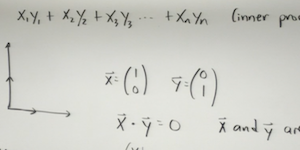### [ view ]

LINEAR SYSTEMS OF EQUATIONS: This lecture provides an introduction to linear systems of equations and vector/matrix manipulations

MATLAB COMMANDS

`n/a`

### Supplementary Videos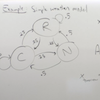View
Matrix Modeling
Code: file.m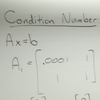View
Condition numbers 1
Code: file.m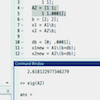View
Condition numbers 2
Code: file.m

### Lecture 2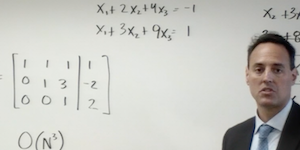### [ view ]

GAUSSIAN ELIMINATION FOR AX=B: We consider the classic solution technique of Gaussian elimination for solving linear systems of equations.

MATLAB COMMANDS

`X=A\B`

### Lecture 3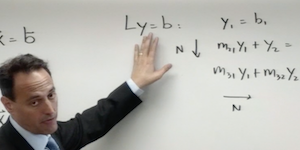### [ view ]

LU MATRIX DECOMPOSITION FOR AX=B: The work horse for solving linear systems is the LU decomposition, which is considered in detail in this lecture.

MATLAB COMMANDS

`LU`

### Supplementary VideosView
Using LU decomposition
Code: file.m